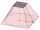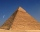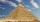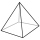# Body volume of a pyramid problems

#### Number of problems found: 61Calculate the volume of a quadrilateral pyramid, the base of which has the shape of a rectangle with dimensions a = 6cm, b = 4cm and height v = 11cm
• Runcated pyramid teapotThe 35 cm high teapot has the shape of a truncated pyramid with the length of the edge of the lower square base a=50 cm and with the edges of the rectangular base b: 20 cm and c: 30 cm. How many liters of water will fit in the teapot?
• The regularThe regular quadrilateral pyramid has a volume of 24 dm3 and a height of 45 cm. Calculate its surface.
• Hexa pyramidThe base of the regular pyramid is a hexagon, which can be described by a circle with a radius of 1 m. Find the volume of the pyramid 2.5 m high.
• A square baseA solid right pyramid has a square base. The length of the base edge is 4 centimeters and the height of the pyramid is 3 centimeters. What is the volume of the pyramid?
• Octagonal pyramidFind the volume of a regular octagonal pyramid with height v = 100 and the angle of the side edge with the plane of the base is α = 60°.
• Tetrahedral pyramidDetermine the surface of a regular tetrahedral pyramid when its volume is V = 120 and the angle of the sidewall with the base plane is α = 42° 30´.
• Cheops pyramidThe Pyramid of Cheops is a pyramid with a square base with a side of 233 m and a height of 146.6 m. It made from limestone with a density of 2.7 g/cm3. Calculate the amount of stone in tons. How many trains with 30 twenty tons wagons carry the stone?
• Secret treasureScouts have a tent in the shape of a regular quadrilateral pyramid with a side of the base 4 m and a height of 3 m. Determine the radius r (and height h) of the container so that they can hide the largest possible treasure.
• Tetrahedral pyramidA regular tetrahedral pyramid is given. Base edge length a = 6.5 cm, side edge s = 7.5 cm. Calculate the volume and the area of its face (side area).
• Right pyramidA right pyramid on a base 4 cm square has a slant edge of 6 cm. Calculate the volume of the pyramid.
• Pyramid heightFind the volume of a regular triangular pyramid with edge length a = 12cm and pyramid height h = 20cm.
• Triangular pyramidWhat is the volume of a regular triangular pyramid with a side 3 cm long?
• Tetrahedral pyramidCalculate the surface S and the volume V of a regular tetrahedral pyramid with the base side a = 5 m and a body height of 14 m.
• Regular triangular pyramidCalculate the volume and surface area of the regular triangular pyramid and the height of the pyramid is 12 centimeters, the bottom edge has 4 centimeters and the height of the side wall is 12 centimetersThe regular quadrangular pyramid has a base length of 6 cm and a side edge length of 9 centimeters. Calculate its volume and surface area.
• Bricks pyramidHow many 50cm x 32cm x 30cm brick needed to built a 272m x 272m x 278m pyramid?
• Pyramid cutWe cut the regular square pyramid with a parallel plane to the two parts (see figure). The volume of the smaller pyramid is 20% of the volume of the original one. The bottom of the base of the smaller pyramid has a content of 10 cm2. Find the area of theGiven is a regular quadrangular pyramid with a square base. The body height is 30 cm and volume V = 1000 cm³. Calculate its side a and its surface area.Calculate the volume of the pyramid with the side 5cm long and with a square base, side-base has angle of 60 degrees.